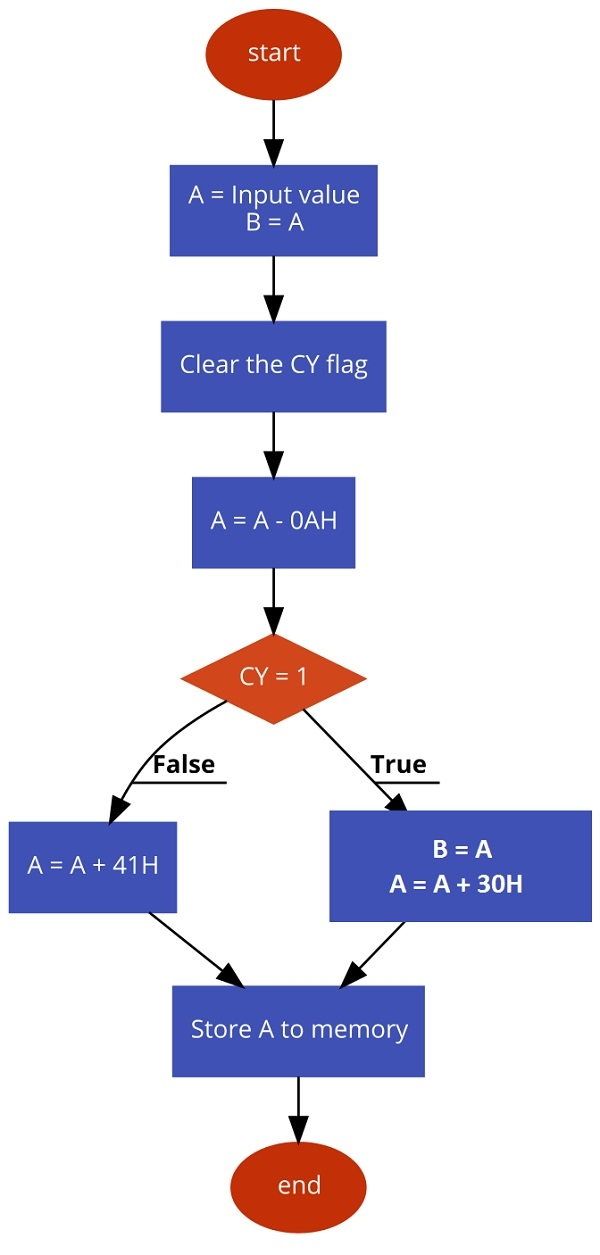# 8085 Program to convert HEX to ASCII

Now let us see a program of Intel 8085 Microprocessor. This program will convert HEX to ASCII values.

## Problem Statement

Write 8085 Assembly language programs to convert Hexadecimal characters to ASCII values.

## Discussion

We know that the ASCII of number 00H is 30H (48D), and ASCII of 09H is39H (57D). So all other numbers are in the range 30H to 39H. The ASCII value of 0AH is 41H (65D) and ASCII of 0FH is 46H (70D), so all other alphabets (B, C, D, E, F) are in the range 41H to 46H.

Here we are providing hexadecimal digit at memory location 8000H, The ASCII equivalent is storing at location 8001H.

The logic behind HEX to ASCII conversion is very simple. We are just checking whether the number is in range 0 – 9 or not. When the number is in that range, then the hexadecimal digit is numeric, and we are just simply adding 30H with it to get the ASCII value. When the number is not in range 0 – 9, then the number is range A – F, so for that case, we are converting the number to 41H onwards.

In the program at first, we are clearing the carry flag. Then subtracting 0AH from the given number. If the value is numeric, then after subtraction the result will be negative, so the carry flag will be set. Now by checking the carry status, we can just add 30H with the value to get ASCII value.

In other hands when the result of the subtraction is positive or 0, then we are adding 41H with the result of the subtraction.

## Input

first input

.
.
.
.
.
.

80000A
.
.
.

.
.
.

second input

.
.
.

.
.
.

800005
.
.
.

.
.
.

third input

.
.
.

.
.
.

80000F
.
.
.

.
.
.

Flow Diagram## Program

F00021,00, 80

F0037E

MOVA, MLoad Acc with the data from memory
F00447

MOVB,ACopy the number into B
F00537

STC
Set Carry Flag
F0063F

CMCComplement Carry Flag
F007D6,0A

SUI0 AHSubtract 0AH from A
F009DA,11, F0

JCNUMWhen the carrier is present, A is numeric
F00CC6,41

F00EC3,14, F0

JMP STOREJumpto store the value
F01178NUMMOVA, BGet back B to A
F012C6

F01423STOREINX HPoint to next location to store address
F01577

MOVM,AStore A to the memory location pointed by HL pair
F01676

HLTTerminate the program

first output

.
.
.

.
.
.

800141
.
.
.

.
.
.

second output

.
.
.

.
.
.

800135
.
.
.

.
.
.

third output

.
.
.
.
.
.
800146
.
.
.
.
.
.